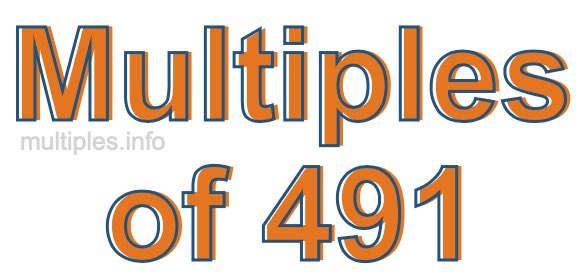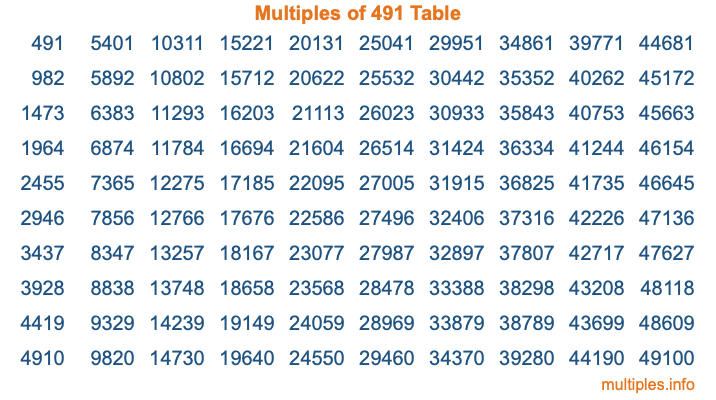Multiples of 491Welcome to the Multiples of 491 page. Here we will first teach you everything you will ever need to know about the multiples of 491, and then give you a study guide summary of everything we taught you to make sure you remember it all. Use this page to look up facts and learn information about the multiples of 491. This page will make you a multiples of four hundred ninety-one expert!

Definition of Multiples of 491
Multiples of 491 are all the numbers that when divided by 491 equal an integer. Each of the multiples of 491 are called a multiple. A multiple of 491 is created by multiplying 491 by an integer.

Therefore, to create a list of multiples of 491, you start with 1 multiplied by 491, then 2 multiplied by 491, then 3 multiplied by 491, and so on for as long as you want. Thus, the list of the first five multiples of 491 is 491, 982, 1473, 1964, and 2455. To see a larger list of multiples of 491, see the printable image of Multiples of 491 further down on this page. We also have a category where you can choose any nth multiple of 491.

Multiples of 491 Checker
The Multiples of 491 Checker below checks to see if any number of your choice is a multiple of 491. In other words, it checks to see if there is any number (integer) that when multiplied by 491 will equal your number. To do that, we divide your number by 491. If the the quotient is an integer, then your number is a multiple of 491.

Is  a multiple of 491?

Least Common Multiple of 491 and ...
A Least Common Multiple (LCM) is the lowest multiple that two or more numbers have in common. This is also called the smallest common multiple or lowest common multiple and is useful to know when you are adding our subtracting fractions. Enter one or more numbers below (491 is already entered) to find the LCM.

Check out our LCM Calculator if you need more details about the Least Common Multiple or if you need the LCM for different numbers for adding and subtraction fractions.

nth Multiple of 491
As we stated above, 491 is the first multiple of 491, 982 is the second multiple of 491, 1473 is the third multiple of 491, and so on. Enter a number below to find the nth multiple of 491.

th multiple of 491

Multiples of 491 vs Factors of 491
491 is a multiple of 491 and a factor of 491, but that is where the similarities end. All postive multiples of 491 are 491 or greater than 491. All positive factors of 491 are 491 or less than 491.

Below is the beginning list of multiples of 491 and the factors of 491 so you can compare:

Multiples of 491: 491, 982, 1473, 1964, 2455, etc.

Factors of 491: 1, 491

As you can see, the multiples of 491 are all the numbers that you can divide by 491 to get a whole number. The factors of 491, on the other hand, are all the whole numbers that you can multiply by another whole number to get 491.

It's also interesting to note that if a number (x) is a factor of 491, then 491 will also be a multiple of that number (x).

Multiples of 491 vs Divisors of 491
The divisors of 491 are all the integers that 491 can be divided by evenly. Below is a list of the divisors of 491.

Divisors of 491: 1, 491

The interesting thing to note here is that if you take any multiple of 491 and divide it by a divisor of 491, you will see that the quotient is an integer.

Multiples of 491 Table
Below is an image of the first 100 multiples of 491 in a table. The table is in chronological order, column by column. The first column has the first ten multiples of 491, the second column has the next ten multiples of 491, and so on.The Multiples of 491 Table is also referred to as the 491 Times Table or Times Table of 491. You are welcome to print out our table for your studies.

Negative Multiples of 491
Although not often discussed or needed in math, it is worth mentioning that you can make a list of negative multiples of 491 by multiplying 491 by -1, then by -2, then by -3, and so on, to get the following list of negative multiples of 491:

-491, -982, -1473, -1964, -2455, etc.

Multiples of 491 Summary
Below is a summary of important Multiples of 491 facts that we have discussed on this page. To retain the knowledge on this page, we recommend that you read through the summary and explain to yourself or a study partner why they hold true.

There are an infinite number of multiples of 491.

A multiple of 491 divided by 491 will equal a whole number.

491 divided by a factor of 491 equals a divisor of 491.

The nth multiple of 491 is n times 491.

The largest factor of 491 is equal to the first positive multiple of 491.

491 is a multiple of every factor of 491.

491 is a multiple of 491.

A multiple of 491 divided by a divisor of 491 equals an integer.

491 divided by a divisor of 491 equals a factor of 491.

Any integer times 491 will equal a multiple of 491.

Multiples of a Number
Here you can get the multiples of another number, all with the same attention to detail as we did for multiples of 491 on this page.

Multiples of
Multiples of 492
Did you find our page about multiples of four hundred ninety-one educational? Do you want more knowledge? Check out the multiples of the next number on our list!